1. General

Regardless of a TSI's angle resolution, accurate angle measurement results are only possible if instrument set up and pointing errors are minimized. Remember that in angle measurement three points are involved: a TSI location and two sighting locations. The two largest errors are instrument centering and pointing. And as with any other measurement type, the more measurements made the greater opportunity for random error cancellation leading to a more accurate result.

2. Centering

The angle desired is the one defined by the ground points. To measure that angle requires an instrument, TSI or sighting mark, be set up at some height above each point. The center of the instrument should be exactly vertically above the ground point but won't be due to random errors. The TSI is oriented using either an optical plummet or plumb bob and, while close, will still have error in it, especially if the optical plummet hasn't been checked. Sighting marks may consist of freely suspended plumb bobs, prism poles with built in bubbles, or tripod/tribrach-mounted target each with its own centering challenge.

Figure E-1 shows the relationship of centering errors on an angle measurement.Figure E-1 Centering Errors

Because these are random errors, they don't behave in a strictly additive process. More on that in a bit.

How can we minimize centering error?

On a TSI the optical plummet should be checked. If it is off, then it should be adjusted or instrument setup should take that into account. Setting up a TSI with a maladjusted optical plummet was discussed in Chapter C. Set Up; Take Down.

For the sighting mark, it depends on the mark's configuration.

* If the mark tribrach-mounted, then the optical plummet must be checked using a plumb bob.
* If the mark is a prism pole, then the rod bubble must be checked and either adjusted or compensated for.
* The least error-prone, from an equipment viewpoint, is a plumb bob. Plumb bob set up error is a function of its suspension: hung from a tripod, held by a person, etc. The more stable the support, the less centering error.

3. Pointing

Pointing error depends on how accurately the TSI operator can sight (point) at the BS and FS marks. A number of things affect this including

* Parallax
* Cross hair design
* Target width
* Target distance

a. Parallax

The effect and removal of parallax been discussed before. By now the operator should be familiar with its behavior and how to remove it. Even though its affect is random, it's an instrumental error which can be adjusted.

b. Cross hair design

In early telescope-based surveying instruments, cross hairs consisted of spider web stands attached to a reticule ring. In addition to their delicacy, they offered the surveyor only a single sighting option: a solid line, Figure E-2(a). As instrumentation and optics evolved, cross hairs eventually became etched lines on optically flat glass plates. Etching allows for more creative and effective cross hair designs, Figures E-2(b) and 2(c).(a)(b)(c) Figure E-2 Cross hair Styles

A narrow sight mark, such as a plumb bob line, at a longer distance may appear narrower than the vertical solid hair causing some pointing error - the hair obscures the string. Using a split hair design, Figure E-2(c), allows the operator to see both the sight mark and the hair. It is often easier to center the mark between hairs than try to make it coincide with a single one, Figures E-3(a) and (b).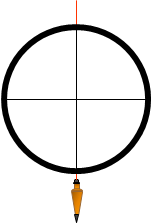(a)(b) Figure E-3 Sighting Plumb Line

c. Target width and distance

Although these are separate characteristics they are related because in combination they affect the sight mark's relative size. Figure E-4 shows the same prism pole sighted at two different distances. The closer the pole is, the more difficult to center the cross hair on it.(a) Distance = x(b) Distance = 2x Figure E-4 Pointing Error

At shorter distances, a narrower sight mark, such as a plumb line, should be used for more accurate pointing.

How much pointing error could we expect? As an extreme, consider sighting the edge of a target rather than its center, Figure E-5.Figure E-5 Sight Distance and Angular Error

Table E-1 shows the error for two different target types at varying distances. The targets are a 0.10 ft diameter prism pole and a 0.03 ft diameter pencil.

 Table E-1 Prism pole Pencil dia = 0.10 ft dia = 0.03 ft Dist (ft) Accy: 1 in Angle, a Accy: 1 in Angle, a 100 2,000 0°01''43" 6,670 0°00'31" 200 4,000 0°00'52" 13,300 0°00'15" 300 6,000 0°00'34" 20,000 0°00'10" 500 10,000 0°00'21" 33,300 0°00'06" 1000 20,000 0°00'10" 66,700 0°00'03"

The accuracy is the distance to the target divided by half the target diameter:Eqn (E-1)

The angle, a, is computed from: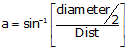Eqn (E-2)

At shorter distances, the error could be quite substantial if the target edge was sighted instead of its center. At those short distances, it wouldn't be very likely that the edge would be sighted but centering the cross hair on a larger target does become less accurate.

The beginning surveyor will notice that angle repetition is harder with larger D/R angle spreads in situations when either or both of the sights are short.

d. Minimizing pointing error

Pointing error is a random error so we can't expect to eliminate it entirely, but it can be minimized with careful target selection and use.

To minimize sighting error, Figure E-6:

* At shorter distances, "finer" targets should be used.

* When using a rigid target that is in contact with the ground mark, such as a prism/range pole or pencil, sight as low on the target as possible.

* When using a plumb bob, sight higher up on the line nearer the support. This is where the line swings the least giving the most repeatable pointing.

* For a tribrach mounted target, sight directly at the target.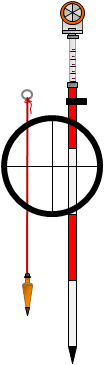Figure E-6 Proper Sighting

What about using the prism glass center where the lines of the individual prisms intersect, Figure E-7? That makes for a nice distinct target, doesn't it?Figure E-7 Sighting Prism Glass

The vertical line passes through the prism lines' intersection (yellow) and the top mount stud (which coincides with the prism pole center).

If the prism is rotated horizontally, the situation in Figure E-8 is created.Figure E-8 Rotated Prism Horizontal Offset

Note how the lines' intersection is offset horizontally. Sighting the intersection introduces a systematic error. The top mount stud is still directly above the ground point so it, or a short barber pole attached to it, should be sighted instead.

4. Combined random errors

(Most of the material in this section has been adapted from Chapter 6 of Adjustment and Computations, first edition, by Wolf & Ghilani).

Many surveyors presume the angle display resolution is the angle measuring accuracy of the TSI. For example, if the TSI display can be set to show angles to 1" then the angle measuring accuracy must be 1". This is no different than a measuring a distance with a tape having a smallest division of 0.01 ft; because you can measure to 0.01 ft doesn't mean your measurement is accurate to 0.01 ft. Holding the 0 end of the tape over the mark will be subject to random error as will reading the tape. Propagating individual random errors will affect the error in the final measurement. Instrument resolution is but one of those errors.

Random errors affecting an angle measurement are can be divided into three categories, Figure E-9:Figure E-9 Centering and Sighting Errors

a. TSI Pointing and Reading error, Epr

This is a characteristic of the instrument itself much like the MSA for electronic distance measurement.

The DIN 18723 standard was developed as a way to express the expected angle uncertainty of a digital instrument. The standard is more a statement of precision rather than accuracy. A later parallel standard, ISO 17123:3, was developed for the same purpose as DIN 18723 but using different calibration criteria. Many instrument manufacturers have adopted the ISO standard in place of the DIN. The instrument manual should show one or the other in the TSI specifications.

The relationship between the DIN 18723 or ISO 17123:3 precision, EDIN, and the expected combined pointing & reading error in a measured angle, Epr, is: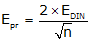Eqn (E-3)

EDIN and Epr are both in seconds of arc.

b. TSI Centering error, Etsi

The error due to TSI centering is: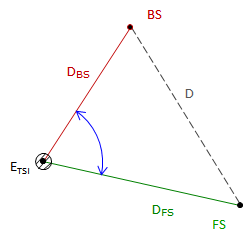Eqn (E-4) Etsi: Angle error due to TSI centering; sec Ei:TSI centering error; linear units DBS, DFS:Distances to BS and FS targets; linear units. D: Distance between BS and FS targets; linear units

Figure E-10
TSI Centuring Error

c. Target Centering error, EtEqn (E-5) Et: Angle error due to target centering; sec EBS, EFS: BS and FS targets centering errors; linear units. DBS, DFS:Distances to BS and FS targets; linear units.
Figure E-11
Target Centering Errors

d. Combined errors

The three errors are propagated as the Error of a Sum to determine the error in the measured angle:Eqn (E-6)

5. Examples

a. Example 1

A TSI is used to measure an angle twice direct and reverse with a resulting average angle of 69°35'47". The BS mark is a tripod mounted target with a centering error of ±0.005 ft. The FS mark is a handheld prism pole. The bottom half of the pole is not visible because of the terrain so a centering error of ±0.02 ft will be used. The TSI has a 01" display and its centering error is ±0.003 ft. From the instrument manual, the TSI's stated ISO 17123:3 angle uncertainty is ±06". Approximate horizontal distances to site marks are shown in Figure E-12.Figure E-12 Example 1

What is the expected error in the measured angle?

TSI Pointing and Reading errorTSI Centering error

To determine this error we need the distance between the targets. This can be computed from the Law of Cosines using the measured angle and distances to the target:thenTarget Centering error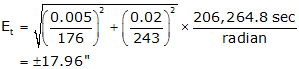CombinedThe expected error in the angle is ±0°00'19"

Analysis

What's the largest source of error? Target centering, the prism pole in particular, is the largest error contributor.

If we replace the pole with a mark similar to that of the BS, the target centering error drops to ±07.23" and expected angular error to ±00°00'10."

b. Example 2

The angle shown in Fig 13 will be measured with a TSI having an ISO 17123:3 angle uncertainty of ±05" and centering error of ±0.003 ft. Both BS and FS marks are tripod mounted, also with a centering error of ±0.003 ft.Figure E-13 Example 2

How many D/R sets must be turned to achieve an expected angular error no greater than ±09"?

The only error affected by the number of angles turned is TSI Pointing and Reading, Eqn (E-3). After substituting TSI Centering and Target Centering errors in Eqn (E-6), it should be solved for the TSI Pointing and Pointing error. Equation (E-3) can then be solved for n.

Compute TSI Centering error, Etsi

Compute distance between targets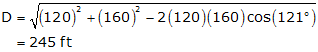compute the errorCompute Target Centering error, Et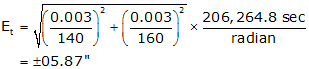Set up Equation (E-6) and solve for nSquare both sides, sort terms, and replace Epr with Eqn (E-3)solve for n:6.49 is the minimum value for n to achieve an expected accuracy of ±09"

n is the number of times the angle is measured, direct or reverse. Since we always measure direct and reverse sets, n must be an even integer. Since it's not possible to measure 6.49/2 = 3.245 D/R sets, n must be 6 (3 D/R) or 8 (4 D/R).

For n=6, the angular error, Eang,  is 09.1" ±juuuust outside the criterion.
For n=8 the angular error is ±08.8".

To meet the ±09" criteria, measure 4 D/R

6. Angle repetition

Measuring the same entity multiple times allows random errors to compensate. Although they can never be entirely eliminated their effect can be lessened through repeated measurements. Measuring an angle direct and reverse is done to compensate for systematic instrumental errors not random errors. The average of a D/R set constitutes a single measurement; it's that resulting angle which has random errors. So to reduce random errors, we repeat D/R sets.

When measuring an angle, once the TSI and targets are set up, their centering error effects do not change during the measurement set. The only thing affecting the final error in the measured angle is the number of times the angle is measured. As seen in the analysis at the end of the second example problem, increasing the repetition from 3 D/R to 4 D/R reduces the expected error from 09.1" to 08.8".

If vary the number of D/R sets in Eqn (E-3) we can see how it affects the TSI Pointing and Reading error. Figure E-14 shows the effect on TSI pointing and reading error as the number of repetitions is increased. Initially, there is a large accuracy improvement up to about 6 D/R. Once past 20 or so sets, additional measurements yield smaller error improvements.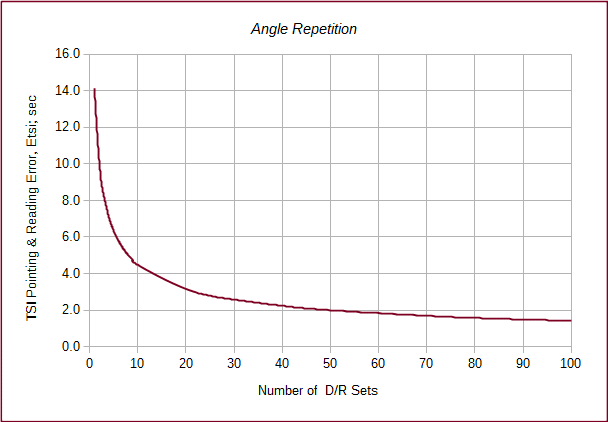Figure E-14 Angle Repetition Effect

Figure E-15 compares three TSIs with different ISO angle uncertainties while varying D/R sets. At fewer D/R sets there is a larger error difference between the TSIs. As the number of D/R sets increase, the error differences become smaller.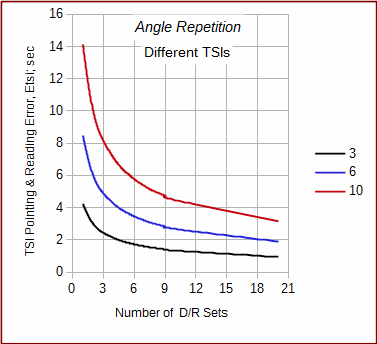Figure E-15 Different TSI Resolutions

Figure E-15 can also be used to determine how mant D/R sets are needed to meet a specific Etsi. For example, to achieve a 4" accuracy:

* the 10" TSI would require 13-14 D/R sets
* the 6" TSI would require 4-5 sets
* the 3" TSI would require 1-2 sets

An example of how this is applied is in the FGCS' Standards and Specifications for Geodetic Control Networks which contains a number of formal standards for angle measurement. In particular, the angle measurement standards for Precise Traverse include these two tables:

Table 2 - Instrumentation Requirements

Order

First

Second

Second

Third

Third

Class

I

II

I

II

Theodolite, least count

0.2"

1.0"

1.0"

1.0"

1.0"

Table 3 - Field Procedures (partial)

Order

First

Second

Second

Third

Third

Class

I

II

I

II

Directions

Number of positions

16

8 or 12†

6 or 8*

4

2

Standard deviation of mean not to exceed

0.4"

0.5"

0.8"

1.2"

2.0"

Rejection limit from the mean.

4"

5"

5"

5"

5"

† 8 if 0.2", 12 if 1.0" resolution.
* 6 if 0.2", 8 if 1.0" resolution.
Theodolite observations for first-order and second-order, class I surveys may be made only at night.

(A position is a single D/R set).

The least count in Table 2 is actually the theodolite reading resolution. Although not the same as the DIN or ISO standard for digital equipment, least count was typically used for the same purposes until the 1980s (the reprint of the Standards was in 1984).

Table 3 contains similar information we divined from Figure E-15 except it includes a bit more detail. It formally identifies the number of D/R sets needed along with some variations based on equipment resolution. As a point of interest, note the night time observation requirement.

Keep in mind that this discussion and Figures E-14 and E-15 address only the TSI Pointing and Reading error. The total expected angle error would also include the TSI Centering and Target Centering errors which are independent of angle repetition.

7. Summary

So what does all this mean?

Because there are so many random error sources in measuring angles we have to be careful with measurement procedure. While equipment quality can make a differnce in angular accuracy, it is only a single contribitor. Equipment centering at all three points (BS, Occupied, FS). target types, and number of measurements all affect the quality of the final result. The discussion and examples in this chapter should provide the surveyor with a picture of how different errors can affect angular accuracy and what steps can be taken to minimize them.

Hits: 9241